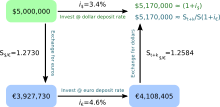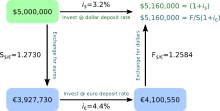Interest rate parity

﻿
Interest rate parity

Interest rate parity is a no-arbitrage condition representing an equilibrium state under which investors will be indifferent to interest rates available on bank deposits in two countries. Two assumptions central to interest rate parity are capital mobility and perfect substitutability of domestic and foreign assets. The interest rate parity condition implies that the expected return on domestic assets will equal the expected return on foreign currency assets, due to an equilibrium in the foreign exchange market resulting from changes in the exchange rate between two countries. Interest rate parity takes on two distinctive forms: uncovered interest rate parity refers to the parity condition in which exposure to exchange rate risk (unanticipated changes in exchange rates) is uninhibited, whereas covered interest rate parity refers to the condition in which a forward contract has been used to cover (eliminate exposure to) exchange rate risk. Each form of the parity condition demonstrates a unique relationship with implications for the forecasting of future exchange rates: the forward exchange rate and the future spot exchange rate.

Assumptions

Interest rate parity rests on certain assumptions, the first being that capital is mobile - investors can readily exchange domestic assets for foreign assets. The second assumption is that assets have perfect substitutability, following from their similarities in riskiness and liquidity. Given capital mobility and perfect substitutability, investors would be expected to hold those assets offering greater returns, be they domestic or foreign assets. However, both domestic and foreign assets are held by investors. Therefore, it must be true that no difference can exist between the returns on domestic assets and the returns on foreign assets. That is not to say that domestic investors and foreign investors will earn equivalent returns, but that a single investor on any given side would expect to earn equivalent returns from either investment decision.

Uncovered interest rate parityA visual representation of uncovered interest rate parity holding in the foreign exchange market, such that the returns from investing domestically are equal to the returns from investing abroad.

When the no-arbitrage condition is satisfied without the use of a forward contract to hedge against exposure to exchange rate risk, interest rate parity is said to be uncovered. Investors are indifferent among the available interest rates in two countries because the exchange rate between those countries is expected to adjust such that the dollar return on dollar deposits is equal to the dollar return on foreign deposits, thereby eliminating the potential for uncovered interest arbitrage profits. Uncovered interest rate parity helps explain the determination of the spot exchange rate. The following equation represents uncovered interest rate parity.$(1 + i_\) = \frac {E(S_{t + k})} {S_t} (1 + i_c)$

where

E(St + k) is the expected future spot exchange rate at time t + k
k is the number of periods into the future from time t
St is the current spot exchange rate at time t
i\$ is the interest rate in the US
ic is the interest rate in a foreign country or currency area (for this example, following a US perspective, it is the interest rate available in the Eurozone)

The dollar return on dollar deposits,$1 + i_\$, is shown to be equal to the dollar return on euro deposits,$\frac {E(S_{t + k})} {S_t} (1 + i_c)$.

Approximation

Uncovered interest rate parity asserts that an investor with dollar deposits will earn the interest rate available on dollar deposits, while an investor holding euro deposits will earn the interest rate available in the eurozone, but also a potential gain or loss on euros depending on the rate of appreciation or depreciation of the euro against the dollar. Economists have extrapolated a useful approximation of uncovered interest rate parity that follows intuitively from these assumptions. If uncovered interest rate parity holds, such that an investor is indifferent between dollar versus euro deposits, then any excess return on euro deposits must be offset by some expected loss from depreciation of the euro against the dollar. Conversely, some shortfall in return on euro deposits must be offset by some expected gain from appreciation of the euro against the dollar. The following equation represents the uncovered interest rate parity approximation.$i_\ = i_c + \frac {{\Delta}E(S_{t + k})} {S_t}$

where

ΔE(St + k) is the change in the expected future spot exchange rate
ΔE(St + k) / St is the expected rate of depreciation of the dollar

A more universal way of stating the approximation is "the home interest rate equals the foreign interest rate plus the expected rate of depreciation of the home currency."

Covered interest rate parityA visual representation of covered interest rate parity holding in the foreign exchange market, such that the returns from investing domestically are equal to the returns from investing abroad.

When the no-arbitrage condition is satisfied with the use of a forward contract to hedge against exposure to exchange rate risk, interest rate parity is said to be covered. Investors will still be indifferent among the available interest rates in two countries because the forward exchange rate sustains equilibrium such that the dollar return on dollar deposits is equal to the dollar return on foreign deposit, thereby eliminating the potential for covered interest arbitrage profits. Furthermore, covered interest rate parity helps explain the determination of the forward exchange rate. The following equation represents covered interest rate parity.$(1 + i_\) = \frac {F_t} {S_t} (1 + i_c)$

where

Ft is the forward exchange rate at time t

The dollar return on dollar deposits,$1 + i_\$, is shown to be equal to the dollar return on euro deposits,$\frac {F_t} {S_t} (1 + i_c)$.

Empirical evidence

Covered interest rate parity (CIRP) is found to hold when there is open capital mobility and limited capital controls, and this finding is confirmed for all currencies freely traded in the present-day. One such example is when the United Kingdom and Germany abolished capital controls between 1979 and 1981. Maurice Obstfeld and Alan Taylor calculated hypothetical profits as implied by the expression of a potential inequality in the CIRP equation (meaning a difference in returns on domestic versus foreign assets) during the 1960s and 1970s, which would have constituted arbitrage opportunities if not for the prevalence of capital controls. However, given financial liberalization and resulting capital mobility, arbitrage temporarily became possible until equilibrium was restored. Since the abolition of capital controls in the United Kingdom and Germany, potential arbitrage profits have been near zero. Factoring in transaction costs arising from fees and other regulations, arbitrage opportunities are fleeting or nonexistent. While CIRP generally holds, it does not hold with precision due to the presence of transaction costs, political risks, tax implications for interest earnings versus gains from foreign exchange, and differences in the liquidity of domestic versus foreign assets.

When both covered and uncovered interest rate parity (UIRP) hold, such a condition sheds light on a noteworthy relationship between the forward and expected future spot exchange rates, as demonstrated below.$UIRP: (1 + i_\) = \frac {E(S_{t + k})} {S_t} (1 + i_c)$$CIRP: (1 + i_\) = \frac {F_t} {S_t} (1 + i_c)$

Dividing the equation for UIRP by the equation for CIRP yields the following equation:$1 = \frac {E(S_{t + k})} {F_t}$

which can be rewritten as:

Ft = E(St + k)

This equation represents the unbiasedness hypothesis, which states that the forward exchange rate is an unbiased predictor of the expected future spot exchange rate. Given strong evidence that CIRP holds, the forward rate unbiasedness hypothesis can serve as a test to determine whether UIRP holds (in order for the forward rate and spot rate to be equal, both CIRP and UIRP conditions must hold). UIRP is found to have some empirical support in tests for correlation between expected rates of currency depreciation and the forward premium or discount. Evidence for the validity and accuracy of the unbiasedness hypothesis, particularly evidence for cointegration between the forward rate and future spot rate, is mixed as researchers have published numerous papers demonstrating both empirical support and empirical failure of the hypothesis.

Wikimedia Foundation. 2010.

Look at other dictionaries:

• interest rate parity — UK US noun [U] FINANCE ► INTEREST PARITY(Cf. ↑interest parity) …   Financial and business terms

• Interest Rate Parity — A theory in which the interest rate differential between two countries is equal to the differential between the forward exchange rate and the spot exchange rate. Interest rate parity plays an essential role in foreign exchange markets, connecting …   Investment dictionary

• Interest rate parity theorem — Interest rate differential between two countries is equal to the difference between the forward foreign exchange rate and the spot rate. The New York Times Financial Glossary …   Financial and business terms

• interest rate parity theorem — Expression that the interest rate differential between two countries is equal to the difference between the forward foreign exchange rate and the spot rate. Bloomberg Financial Dictionary …   Financial and business terms

• Uncovered Interest Rate Parity - UIP — A parity condition stating that the difference in interest rates between two countries is equal to the expected change in exchange rates between the countries’ currencies. If this parity does not exist, there is an opportunity to make a… …   Investment dictionary

• Covered Interest Rate Parity — This term refers to a condition where the relationship between interest rates and the spot and forward currency values of two countries are in equilibrium. As a result, there are no interest rate arbitrage opportunities between those two… …   Investment dictionary

• Covered Interest Rate Parity — The principle that the yields from interest bearing foreign and domestic investments should be equal when the currency market is used to predetermine the domestic currency payoff from a foreign investment. Bloomberg Financial Dictionary …   Financial and business terms

• Interest Rate Differential - IRD — A differential measuring the gap in interest rates between two similar interest bearing assets. Traders in the foreign exchange market use interest rate differentials (IRD) when pricing forward exchange rates. Based on the interest rate parity, a …   Investment dictionary

• Interest rate cap and floor — Interest rate c An interest rate cap is a derivative in which the buyer receives payments at the end of each period in which the interest rate exceeds the agreed strike price. An example of a cap would be an agreement to receive a payment for… …   Wikipedia

• Net Interest Rate Differential — In international markets, the difference in the interest rates of two distinct economic regions. If a trader is long the NZD/USD pair, he or she owns the New Zealand currency and borrows the US currency. These New Zealand dollars can be placed… …   Investment dictionary